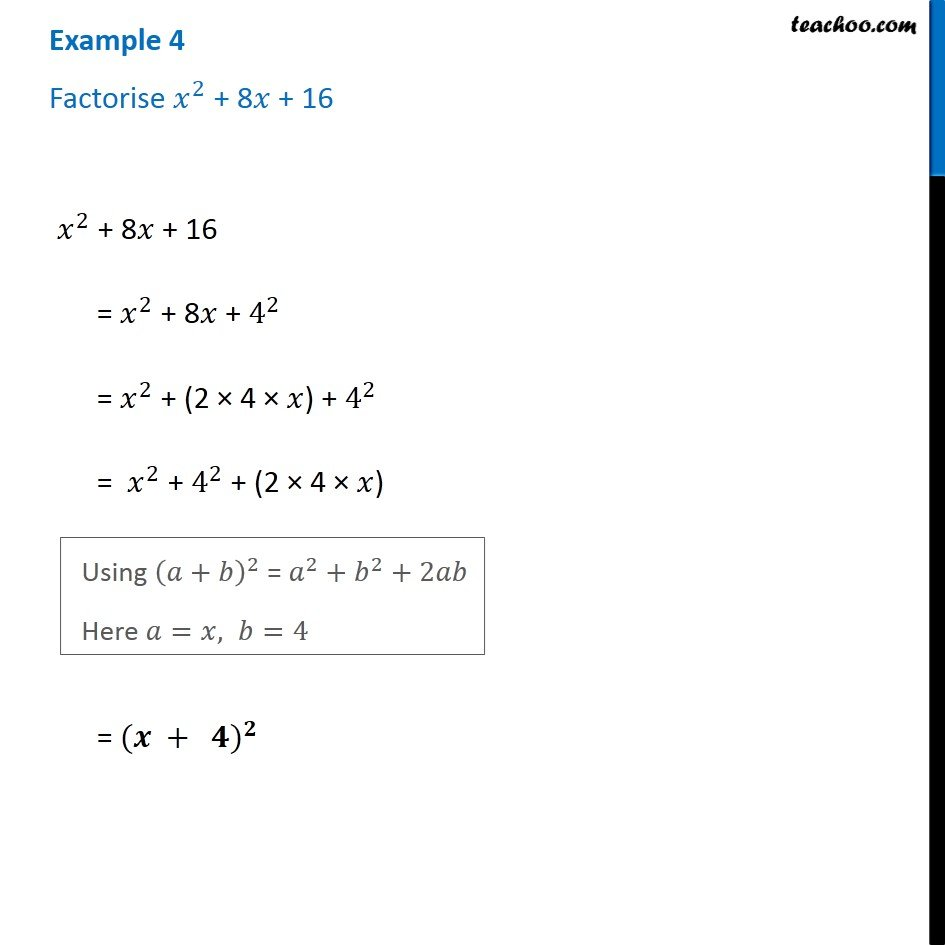1. Chapter 14 Class 8 Factorisation
2. Serial order wise
3. Examples

Transcript

Example 4 Factorise 𝑥^2 + 8𝑥 + 16 𝑥^2 + 8𝑥 + 16 = 𝑥^2 + 8𝑥 + 4^2 = 𝑥^2 + (2 × 4 × 𝑥) + 4^2 = 𝑥^2 + 4^2 + (2 × 4 × 𝑥) Using (𝑎+𝑏)^2 = 𝑎^2+𝑏^2+2𝑎𝑏 Here 𝑎=𝑥, 𝑏=4 = (〖𝒙 + 𝟒)〗^𝟐